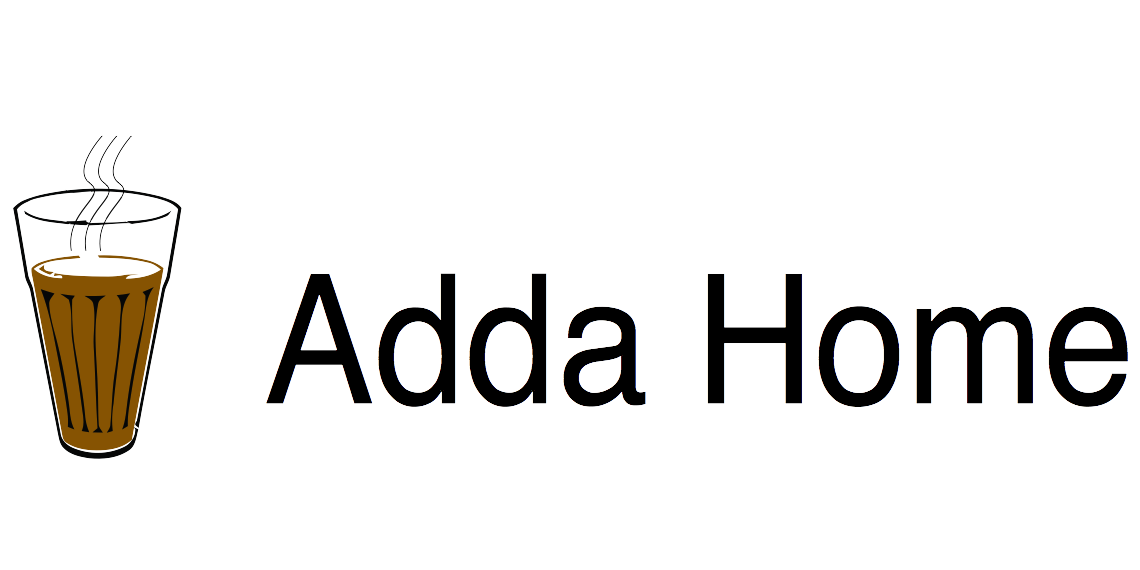# How e-litmus marks are calculated?

i attempted 16 question .out of 16 question i am 100% sure about 12 question. i think my score is calculted incorrect.
quant-26.30
problem solving-30
englsih-56.30

Negative marking starts once more than 25% of your attempted questions are incorrect.
This is separately calculated for each sections.

How many did you attempt section wise?

I am guessing you performed -

Quant 3/5

1. 30 marks for 3 correct answers.
2. Allowed incorrect = 1.25 = 25% of 5.
3. Your incorrect = 2 = 40%
4. Negative marking on (2 - 1.25) = 0.75.
5. Penalty = -5
6. Total deducted = -5 x 0.75 = -3.75.
7. Final score = 30 - 3.75 = 26.25 => Rounded to 26.30

Similarly you can calculate for other sections -
Logical 3/3 = 30
Verbal 6/9 = 56.30

1 Like

no
i have attempted
quant -4
proble solving-4
and verbel -8
but i unbale to understand how my marks are calculted.

As I mentioned above.
For each correct answer you get +10.
You are allowed wrong answers as long as they are less than 25% of your attempted questions. Keep in mind that these are calculated separately for sections.

Suppose you attempted 10 questions in total.
So 25% means you can get at most 2 answers wrong.

You get negative marking only for portion outside of allowed.

Cases -

1. 10/10 correct - Score = 100
2. 9/10 correct - Score = 90
3. 8/10 correct - Score = 80
4. 7/10 correct - Score = 67.5 ( Allowed mistakes is 2.5, you got 3 wrong so negative marking only on the 0.5 extra wrong. So, penalty is 2.5 marks.
5. 6/10 correct - Score = 52.5

Formula

(Correct x 10) - (( Incorrect - 25 % of Attempted ) x 5)

bro you did not get what i am asking !!!
i attempted 4 question in quant in which 3(q1,q2,q3) are right. and q5 is wrong

i.e
according to formula
3*10- ((1-25into4/100)*5) =30 ( not you mentioned 26.30)

i attempted 8 question in english in which 6 (q41,q43,q44,q54,q56,q58)are right.
6*10-((2-25into8/100)*5)=60 (not you entioned 56.30)

just like in problem solving
i attempted 4 question (3 crypto arithetic and 1 data sufficiency which is wrong )
so
3*10- ((1-25into4/100)*5) =30 .

1 Like

Actually I don’t know the exact formula. I read about the marking scheme in a lot of articles and formulated as above.
Maybe the real marking algorithm is different.

As per my score my formula is correct. Also I could calculate your score accurately.

But maybe it is wrong. We can’t do anything about it.
eLitmus does not review score.
It has also happened before that many people got 0 in Verbal and they could do nothing about it.

Then e -litmus doing it but knowingly.
i can not give ph test again and again , i do not have money.
should i complain it into consumer court ???

Try calling eLitmus support and talk to them before taking a big step.
I have heard about wrong score but never knew the complete story.

1 Like